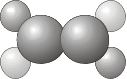# 3: Symmetry Classification of Molecules: Point Groups

•• Contributed by Claire Vallance
• Professor of Physical Chemistry (Department of Chemistry) at University of Oxford

It is only possible for certain combinations of symmetry elements to be present in a molecule (or any other object). As a result, we may group together molecules that possess the same symmetry elements and classify molecules according to their symmetry. These groups of symmetry elements are called point groups (due to the fact that there is at least one point in space that remains unchanged no matter which symmetry operation from the group is applied). There are two systems of notation for labeling symmetry groups, called the Schoenflies and Hermann-Mauguin (or International) systems. The symmetry of individual molecules is usually described using the Schoenflies notation, and we shall be using this notation for the remainder of the course1.

Some of the point groups share their names with symmetry operations, so be careful you do not mix up the two. It is usually clear from the context which one is being referred to.

## Molecular Point Groups

1. $$C_1$$ - contains only the identity (a $$C_1$$ rotation is a rotation by 360° and is the same as the identity operation $$E$$) e.g. CHDFCl.1. $$C_i$$ - contains the identity $$E$$ and a center of inversion $$i$$.1. $$C_S$$ - contains the identity $$E$$ and a plane of reflection $$\sigma$$.1. $$C_n$$ - contains the identity and an $$n$$-fold axis of rotation.1. $$C_{nv}$$ - contains the identity, an $$n$$-fold axis of rotation, and $$n$$ vertical mirror planes $$\sigma_v$$.1. $$C_{nh}$$ - contains the identity, an $$n$$-fold axis of rotation, and a horizontal reflection plane $$\sigma_h$$ (note that in $$C_{2h}$$ this combination of symmetry elements automatically implies a center of inversion).1. $$D_n$$ - contains the identity, an $$n$$-fold axis of rotation, and $$n$$ 2-fold rotations about axes perpendicular to the principal axis.1. $$D_{nh}$$ - contains the same symmetry elements as $$D_n$$ with the addition of a horizontal mirror plane.1. $$D_{nd}$$ - contains the same symmetry elements as $$D_n$$ with the addition of $$n$$ dihedral mirror planes.1. $$S_n$$ - contains the identity and one $$S_n$$ axis. Note that molecules only belong to $$S_n$$ if they have not already been classified in terms of one of the preceding point groups (e.g. $$S_2$$ is the same as $$C_i$$, and a molecule with this symmetry would already have been classified).

The following groups are the cubic groups, which contain more than one principal axis. They separate into the tetrahedral groups ($$T_d$$, $$T_h$$ and $$T$$) and the octahedral groups ($$O$$ and $$O_h$$). The icosahedral group also exists, but is not included below.

1. $$T_d$$ - contains all the symmetry elements of a regular tetrahedron, including the identity, 4 $$C_3$$ axes, 3 $$C_2$$ axes, 6 dihedral mirror planes, and 3 $$S_4$$ axes e.g. $$CH_4$$.1. $$T$$ - as for $$T_d$$ but no planes of reflection.
2. $$T_h$$ - as for $$T$$ but contains a center of inversion.
3. $$O_h$$ - the group of the regular octahedron e.g. $$SF_6$$.1. $$O$$ - as for $$O_h$$, but with no planes of reflection.

The final group is the full rotation group $$R_3$$, which consists of an infinite number of $$C_n$$ axes with all possible values of $$n$$ and describes the symmetry of a sphere. Atoms (but no molecules) belong to $$R_3$$, and the group has important applications in atomic quantum mechanics. However, we won’t be treating it any further here.

Once you become more familiar with the symmetry elements and point groups described above, you will find it quite straightforward to classify a molecule in terms of its point group. In the meantime, the flowchart shown below provides a step-by-step approach to the problem.1Though the Hermann-Mauguin system can be used to label point groups, it is usually used in the discussion of crystal symmetry. In crystals, in addition to the symmetry elements described above, translational symmetry elements are very important. Translational symmetry operations leave no point unchanged, with the consequence that crystal symmetry is described in terms of space groups rather than point groups.